# DAV Class 8 Maths Chapter 11 Worksheet 3 Solutions

The DAV Maths Class 8 Solutions and DAV Class 8 Maths Chapter 11 Worksheet 3 Solutions of Understanding Quadrilaterals offer comprehensive answers to textbook questions.

## DAV Class 8 Maths Ch 11 WS 3 Solutions

Question 1.
ABCD is a quadrilateral in which the diagonals are equal and bisect each other at right angles. What can you say about the figure? Is it (i) a rectangle (ii) a rhombus (iii) a square?
Solution: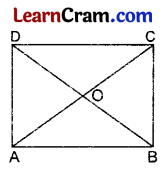$$\overline{\mathrm{AC}}=\overline{\mathrm{BD}}$$
$$\overline{\mathrm{AC}}$$ bisects BD at 90° [Given]
∴ It is a square.
[∵ If the diagonals are equal and bisect each other at 90° then it is a square]
Hence ABCD is a square.Question 2.
The diagonals of a rhombus are in the ratio 5 : 12. If its perimeter is 104 cm, find the lengths of the sides of the diagonals.
Solution: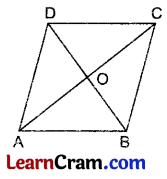The perimeter of the rhombus = 104 cm
∴ One side AB = $$\frac{104}{2}$$ = 52 cm
Let $$\overline{\mathrm{BD}}$$ be 5x cm and $$\overline{\mathrm{AC}}$$ be 12x cm.
∴ $$\overline{\mathrm{OA}}=\frac{1}{2} \overline{\mathrm{AC}}$$
= $$\frac{1}{2}$$ × 12x
= 6x cm
$$\overline{\mathrm{OB}}=\frac{1}{2} \overline{\mathrm{BD}}$$
= $$\frac{1}{2}$$ × 5x
= $$\frac{5x}{2}$$ cm
In rt. ΔAOB,
OA2 + OB2 = AB2 [From Pythagoras Theorem]
⇒ (6x)2 + ($$\frac{5x}{2}$$)2 = (52)2
⇒ 36x2 + $$\frac{25}{4} x^2$$ = 2704
⇒ $$\frac{169}{4} x^2$$ = 2704
⇒ x2 = $$\frac{2704 \times 4}{169}$$
⇒ x2 = 64
⇒ x = 8
Hence the length of the diagonals is 12 × 8 = 96 cm and 5 × 8 = 40 cm.

Question 3.
A pair of adjacent sides of a rectangle are in the ratio 3 : 4. If its diagonal is 20 cm, find the lengths of the sides and hence, the perimeter of the rectangle.
Solution: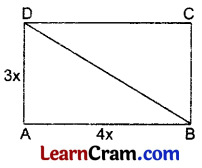Let the two adjacent sides be 4x cm and 3x cm.
∴ $$\overline{\mathrm{AB}}$$ = 4x cm and $$\overline{\mathrm{AD}}$$ = 3x cm
Diagonal BD = 20 cm
∴ In the right ∆DAB,
AB2 + AD2 = BD2 [From Pythagoras Theorem]
⇒ (4x)2 + (3x)2 = (20)2
⇒ 16x2 + 9x2 = 400
⇒ 25x2 = 400
⇒ x2 = 16
⇒ x = 4
∴ Length of the sides are 4 × 4 = 16 cm and 4 × 3 = 12 cm
Hence the perimeter = 2[l + b]
= 2[16 + 12]
= 56 cm.Question 4.
ABCD is a rhombus whose diagonals intersect at O. Show that ∆AOB ≅ ∆COD.
Solution: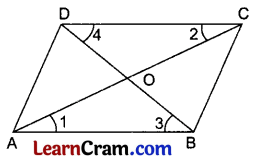In ∆AOB and ∆COD
∠1 = ∠2 [Alternate angles]
∠3 = ∠4 [Alternate angles]
$$\overline{\mathrm{AB}}=\overline{\mathrm{DC}}$$ [Sides of a rhombus]
∴ ∆AOB ≅ ∆COD [ASA]

Question 5.
The diagonals of a rectangle ABCD intersect at O. If ∠BOC = 70°, find ∠ODA.
Solution: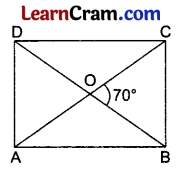∠AOD = ∠BOC [Vertically opposite angles]
∴ ∠AOD = 70° [Given]
∠ODA = ∠OAD = x [Let]
[Angles opposite to equal sides of a ∆ are also equal]
∴ In ∆AOD
∠x + ∠x + 70° = 180° [Angle sum property of a ∆]
⇒ 2∠x = 180° – 70°
⇒ 2∠x = 110°
⇒ ∠x = 55°
Hence ∠ODA = 55°.

Question 6.
ABC and ADC are two equilateral triangles on a common base AC. Find the angles of the resulting quadrilateral. Show that it is a rhombus.
Solution: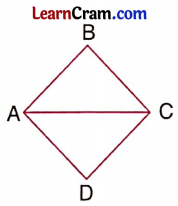∆ABC is an equilateral triangle. [Given]
∴ AC = AB = AC ……(i)
[Property of equilateral triangle]
AC = AD = DC …….(ii)
From (i) and (ii) we get,
AB = BC = CD = DA
Hence ABCD is a rhombus.Question 7.
ABCD is a rectangle in which $$\overline{\mathrm{DP}}$$ and $$\overline{\mathrm{BQ}}$$ are perpendiculars from D and B respectively on diagonal $$\overline{\mathrm{AC}}$$. Show that
(iii) $$\overline{\mathrm{DP}}=\overline{\mathrm{BQ}}$$
Solution: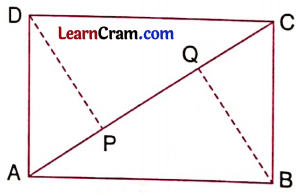$$\overline{\mathrm{AD}}=\overline{\mathrm{BC}}$$ [Opposite sides of a rectangle]
∠DPA = ∠BQC = 90° [Given]
∠DAP = ∠BCQ [Alternate angles]
∴ ∆ADP ≅ ∆CBQ [By AAS Property]
(iii) $$\overline{\mathrm{DP}}=\overline{\mathrm{BQ}}$$ [C.P.C.T.]

### DAV Class 8 Maths Chapter 11 Value Based Questions

Question 1.
There was a wasteland near a locality in the shape of a parallelogram ABCD. The residents of the locality, with the permission of concerned authorities decided to fence the land and convert it into a park with shady trees, plants, and a small herbal garden. The perimeter of the land is 320 m. Length AB of the land is 100 m. The fencing has to be done only along sides AB, BC, and CD of the land. AD will be used as a gate.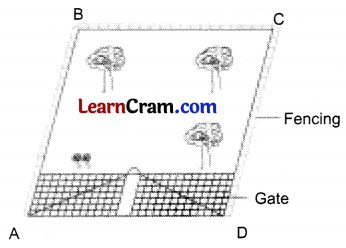(i) Find the cost of fencing the land at ₹ 26 per meter.
(ii) Do you like the idea of the residents converting Wasteland into a park? Why?
(iii) In what other ways can you make use of wasteland?
Solution:
(i) Given: Perimeter of the land = 320 m
Length AB = 100 m
Let the length BC be x m.
Then, AB + BC + CD + AD = 320 m
⇒ 100 + x + 100 + x = 320 m (∵ Opposite sides of a parallelogram are equal)
⇒ 200 + 2x = 320 m
⇒ 2x = 120 m
⇒ x = 60 m
∴ The length of fencing = AB + BC + CD
= 100 + 60 + 100
= 260 m
Cost of the fencing land = ₹ 26 × 260 = ₹ 6760

(ii) Yes, we like the idea of the residents converting wasteland into a park. Because, by doing so, we can get fresh air and medicinal plants. We can also do exercise in such a healthy atmosphere.

(iii) We can use the wasteland to make orphanages, hospitals, schools, and/or yoga centers.